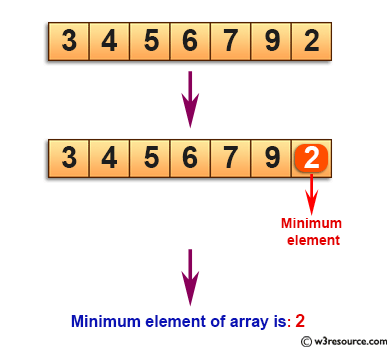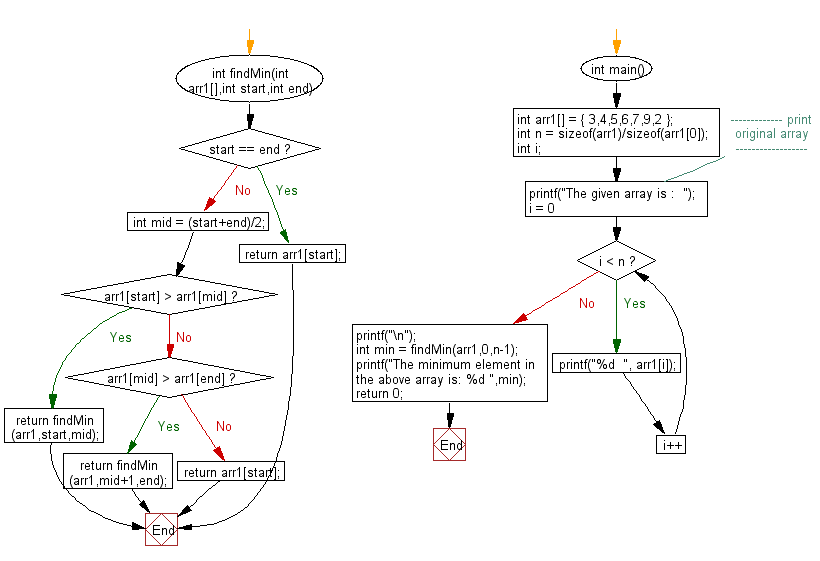﻿ C exercises: Find minimum element in a sorted and rotated array - w3resource# C Exercises: Find minimum element in a sorted and rotated array

## C Array: Exercise-57 with Solution

Write a program in C to find minimum element in a sorted and rotated array.

Pictorial Presentation:Sample Solution:

C Code:

``````#include <stdio.h>

int findMin(int arr1[],int start,int end)
{
if(start == end)
{
return arr1[start];
}
int mid = (start+end)/2;
if(arr1[start] > arr1[mid])
{
return findMin(arr1,start,mid);
}
else
if(arr1[mid] > arr1[end])
{
return findMin(arr1,mid+1,end);
}
else
{
return arr1[start];
}
}

int main()
{
int arr1[] = { 3,4,5,6,7,9,2 };
int n = sizeof(arr1)/sizeof(arr1);
int i;
//------------- print original array ------------------
printf("The given array is :  ");
for(i = 0; i < n; i++)
{
printf("%d  ", arr1[i]);
}
printf("\n");
//------------------------------------------------------
int min = findMin(arr1,0,n-1);
printf("The minimum element in the above array is: %d ",min);
return 0;
}
```
```

Sample Output:

```The given array is :  3  4  5  6  7  9  2
The minimum element in the above array is: 2
```

Flowchart:C Programming Code Editor:

Improve this sample solution and post your code through Disqus.

What is the difficulty level of this exercise?

﻿

## C Programming: Tips of the Day

Where is the C auto keyword used?

auto is a modifier like static. It defines the storage class of a variable. However, since the default for local variables is auto, you don't normally need to manually specify it.

Ref : https://bit.ly/3yzwC9r